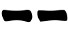## Thursday 2 June 2016A simple method for casting hexagrams using playing cards has been devised by Russel Cottrell and described on his site (which is full of intereseting methods I will investigate and review in the future). Have a look at his site if you haven't done it yet.

It proceeds as follows:
1. Shuffle the deck;
2. Pick 6 card and lay them facing down in a column
3. Turn the cards one by one (from bottom to top) :
• If it's a black face card, draw;
• If it's a black pip card, draw;
• If it's a red pip card, draw;
• If it's a red face card, draw;
The picture on the right shows an example of this method.

#### Probabilities

A first approximation could be:
Prob(6) = Prob(9) = 6/52  = 11.54%
Prob(8) = Prob(7) = 20/52 = 38.46%
Prob(yin) = Prob(yang) = 1/2
but this is correct only if the cards were extracted one at the time and reinserted in the deck before extracting the next one. The probabilities above are close to the three coins method up to 1%. Probabilities would be exactly the same as the three coins method if we removed one pip card for each suit, as Russel suggests.

A full analysis of the method as described above would be rather long: the probabilities would be different for each line meaing there would be a lot of conditional probabilites to take into consideration. I'll surely work on it in the future.

The only thing I want to point out here is that the method has lower probability for moving lines. Considering the extreme case of having 6 moving lines of the same type (i.e. 1>2 or 2>1), the probability is almost 100 times lower with this method than with the three coins method:

Cards images: Atlasnye